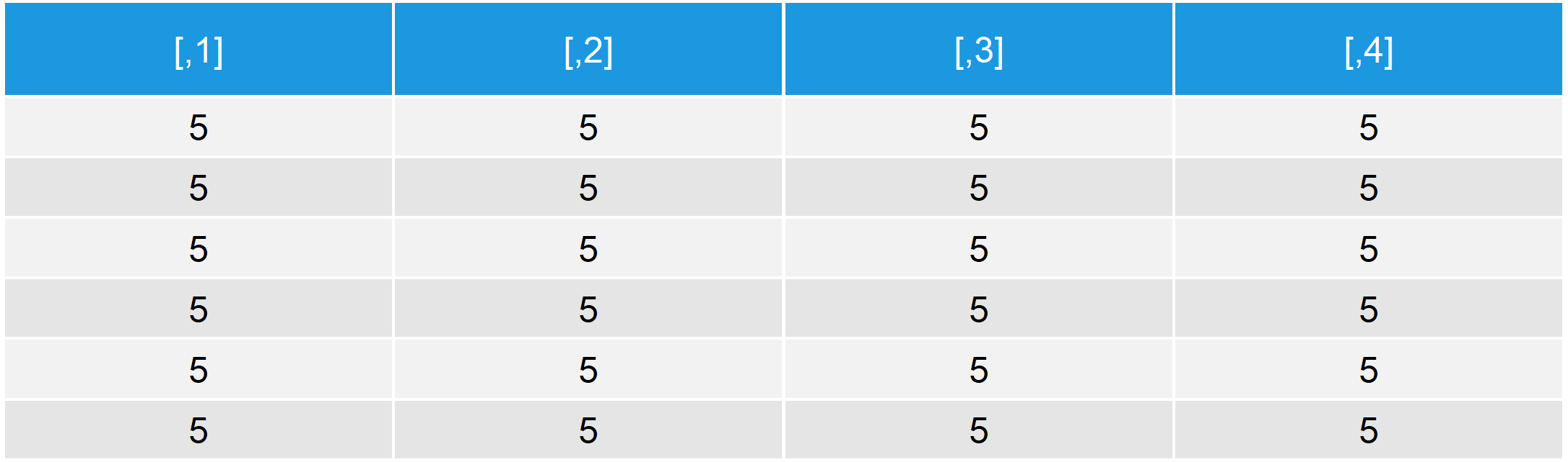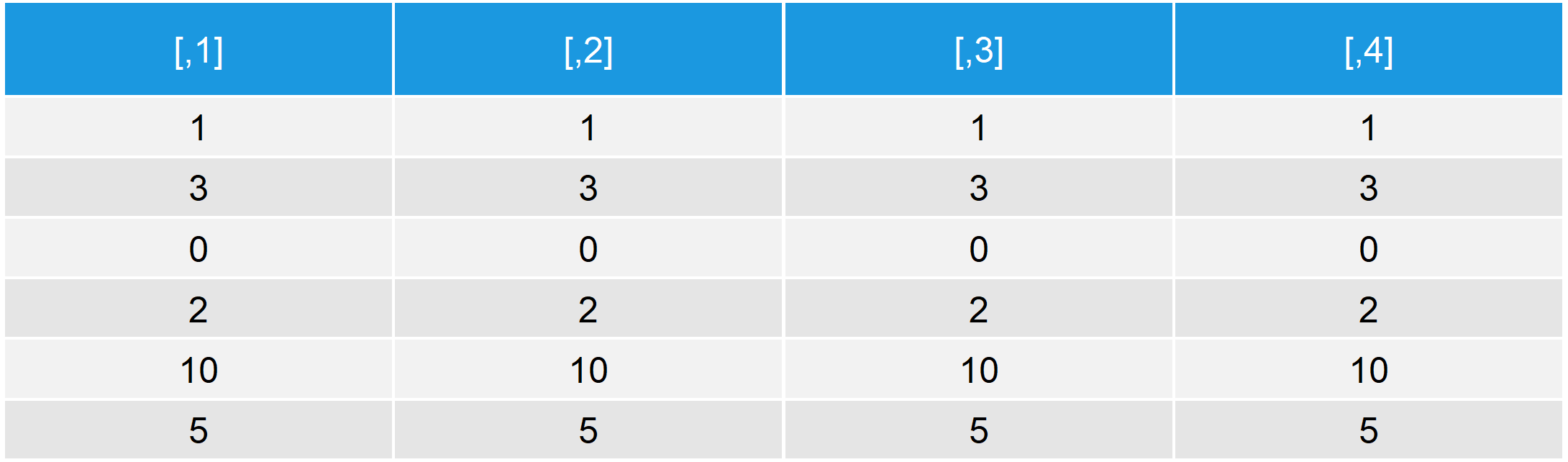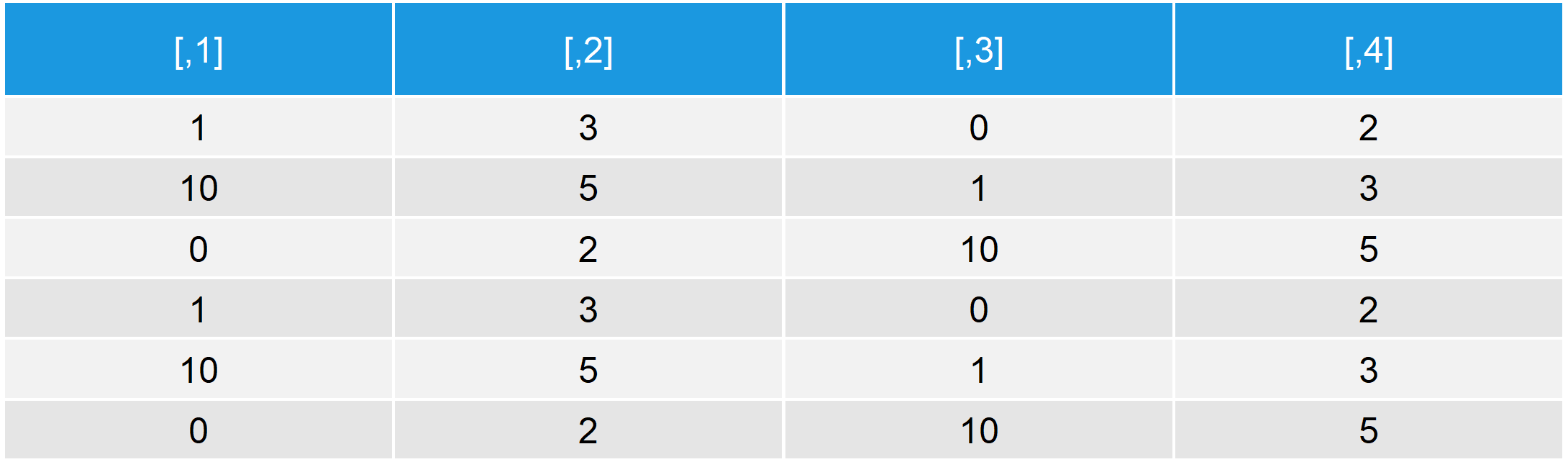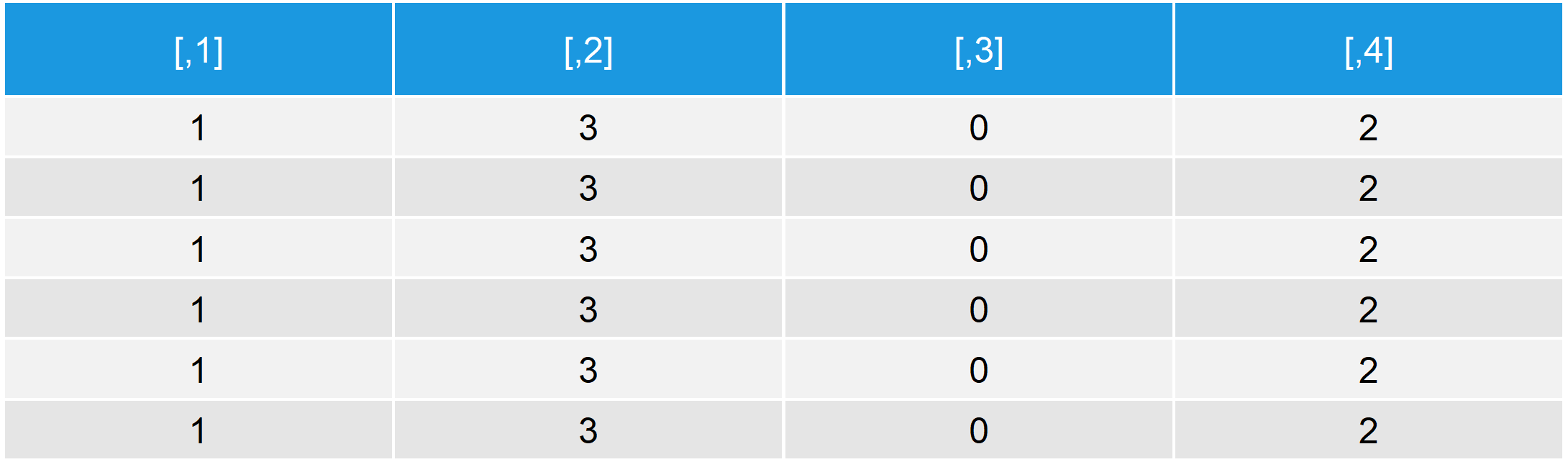# R sweep Function | 3 Example Codes (Matrix Operation with MARGIN & STATS)

Basic R Syntax:

`sweep(x = data, MARGIN = 1, STATS = 1, FUN = "+")`

The sweep R function applies an operation (e.g. + or -) to a data matrix by row or by column.

The following parameters have to be specified within the sweep function:

• x: Typically a matrix.
• MARGIN: Specifies typically whether the operation should be applied by row or by column. MARGIN = 1 operates by row; MARGIN = 2 operates by column.
• STATS: Specifies usually the value that should be used for the operation (e.g. the value that should be added or subtracted).
• FUN: The operation that should be carried out (e.g. + or -).

Further (not necessarily needed) arguments can be specified for sweep in R. Type ?sweep into your RStudio console to learn more.

However, let’s not waste too much time and let’s dive strictly into the examples…

## Example 1: Sweep Matrix in R

```data <- matrix(0, nrow = 6, ncol = 4)                          # Create example matrix
data                                                           # Print matrix to R console```Table 1: Example Matrix for the Application of sweep in R.

We have created a matrix with 4 columns and 6 rows; All values are zero.

Now, let’s apply the R sweep command to this example matrix:

```data_ex1 <- sweep(x = data, MARGIN = 1, STATS = 5, FUN = "+")  # Apply sweep in R
data_ex1                                                       # Print example 1 to console```Table 2: Example Matrix After Simple Application of sweep in R.

As you can see, all zeros where replaced by 5. But why? Let’s go through the code step by step:

• x: Our example matrix, which is called data.
• MARGIN: We want to apply the operation by row. Therefore, we set MARGIN = 1.
• STATS: In our operation, we want to use the value 5 for each data cell.
• FUN: We want to apply the operation plus.

To explain it as simple as possible: With the previous code, we added the value 5 to each of our data cells.

Sounds stupid? Let’s move on to a more realistic example…

## Example 2: Apply sweep() with Complex Specification of STATS

For the next example, I’m going to use the same example data set as in Example 1 (our matrix with zeros). However, this time I’m going to specify the STATS argument in a more complex way. The rest of the code is kept as in Example 1:

```data_ex2 <- sweep(x = data, MARGIN = 1,                        # Sweep with Complex STATS
STATS = c(1, 3, 0, 2, 10, 5), FUN = "+")
data_ex2                                                       # Print example 2 to console```Table 3: Example Matrix after Applying sweep with Complex STATS Specification.

All rows are different. So what happened this time?!

By using a vector with six different numbers for the STATS argument (i.e. c(1, 3, 0, 2, 10, 5)), we can use a different value for each of our six rows. We added the value 1 to each cell of the first row, 3 to each cell of the second row, 0 to each cell of the third and so on…

So, what about the MARGIN argument? You guessed it – That’s what I’m going to show you now…

## Example 3: The MARGIN Argument of the Sweep R Function

For the third example, I’m keeping the code exactly as in Example 2, but this time I’m going to change the specification of MARGIN:

```data_ex3 <- sweep(x = data, MARGIN = 2,                        # Change MARGIN Argument to 2
STATS = c(1, 3, 0, 2, 10, 5), FUN = "+")```

Oh gosh, what happened?!!

Warning message:
In sweep(x = data, MARGIN = 2, STATS = c(1, 3, 0, 2, 10, 5), FUN = “+”) :
STATS is longer than the extent of ‘dim(x)[MARGIN]’

Let’s have a look at the output:

`data_ex3                                                       # Print example 3 to RStudio`Table 4: Warning: STATS is longer than the extent of ‘dim(x)[MARGIN]’.

As you can see, we received a valid output. However, the operation recycled across the end of each row. Usually you should try to avoid this by specifying the length of STATS equal to the number of rows/columns.

Let’s do this:

```data_ex3_b <- sweep(x = data, MARGIN = 2,                      # Change length of STATS
STATS = c(1, 3, 0, 2), FUN = "+")
data_ex3_b                                                     # Print example 3b to RStudio```Table 5: Fitting Length of STATS Argument.

After deleting the last two values of our STATS specification (i.e. 10 and 5), the output data is ordered well.

Looks much better!

## Video Explanation: sweep in R

In case you need further explanations on the code of this page, you may check out the following YouTube video on the Statistics Globe YouTube channel. It explains the syntax of this article in some more detail:

Please accept YouTube cookies to play this video. By accepting you will be accessing content from YouTube, a service provided by an external third party.If you accept this notice, your choice will be saved and the page will refresh.

More examples? I know, the sweep function is not easy to understand. Have a look at the following video of the R programming Library YouTube channel:

Please accept YouTube cookies to play this video. By accepting you will be accessing content from YouTube, a service provided by an external third party.If you accept this notice, your choice will be saved and the page will refresh.

Subscribe to the Statistics Globe Newsletter

•September 16, 2019 7:07 pm

Great article!

•September 17, 2019 5:51 am

•Amy
November 15, 2019 9:33 pm

Really helpful, and well explained. Thank-you

•November 18, 2019 8:17 am

Thank you Amy 🙂

•Unnikrishnan C
January 6, 2021 11:45 pm

Sweep is not difficult to understand. But could you please illustrate a practical situation where it is used. This will go a long way to make it more memorable for the learner.

•January 7, 2021 7:10 am

Hey Unnikrishnan,

Thanks for another nice comment!

In fact, I’m not using sweep often myself. However, I found a nice thread on Stack Overflow, where people report about how they use the sweep function.

For instance, people use it to compute weighted covariance matrices or weighted sums.

Best regards,

Joachim